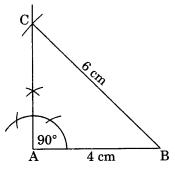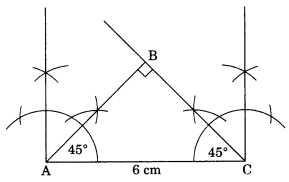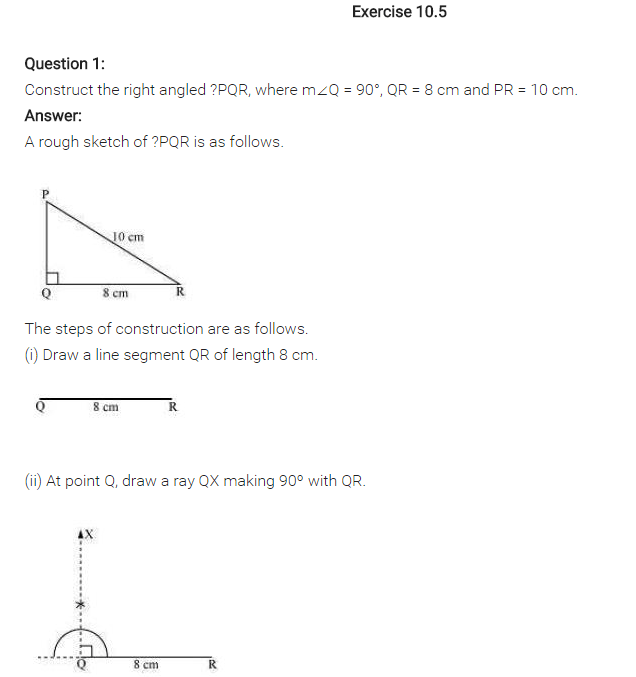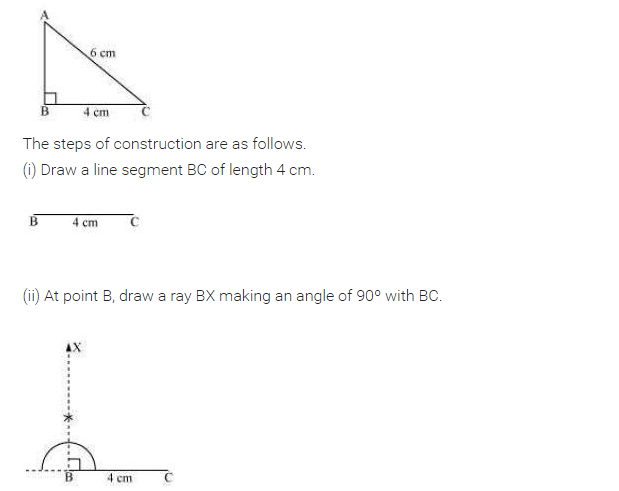# NCERT Solutions for Class 7 Maths Chapter 10 Practical Geometry Ex 10.5

NCERT Solutions for Class 7 Maths Chapter 10 Practical Geometry Ex 10.5

### NCERT Solutions for Class 7 Maths Chapter 10 Practical Geometry Ex 10.5

NCERT Solutions for Class 7 Maths Chapter 10 Practical Geometry Exercise 10.5
Ex 10.5 Class 7 Maths Question 1.
Construct the right angled ∆PQR, where m∠Q = 90°, QR = 8 cm and PR = 10 cm.Solution:
Steps of construction:
(i) Draw QR = 8 cm.
(ii) Draw m∠Q = 90°.
(iii) Draw an arc with centre R and radius 10 cm to cut the perpendicular line at P.
(iv) ∆PQR is the required triangle.
[Using RHS criterion]

Ex 10.5 Class 7 Maths Question 2.
Construct a right-angled triangle whose hypotenuse is 6 cm long and one of the legs is 4 cm long.Solution:
Steps of construction:
(i) Draw AB = 4 cm.
(ii) Draw ∠A = 90°.
(iii) Cut BC = 6 cm.
(iv) ∆CAB is the required right-angled triangle.
[Using RHS criterion]

Ex 10.5 Class 7 Maths Question 3.
Construct an isosceles right-angled triangle ABC, where m∠ACB = 90° and AC = 6 cm.Solution:
Steps of construction:
(i) Draw AC = 6 cm.
(ii) Draw ∠A =∠C = 45° to meet each other at B such that m∠B = 90°.
(iii) ∆ABC is right-angled isosceles triangle which is the required triangle.
[Using RHS criterion]## SabDekho

The Complete Educational Website